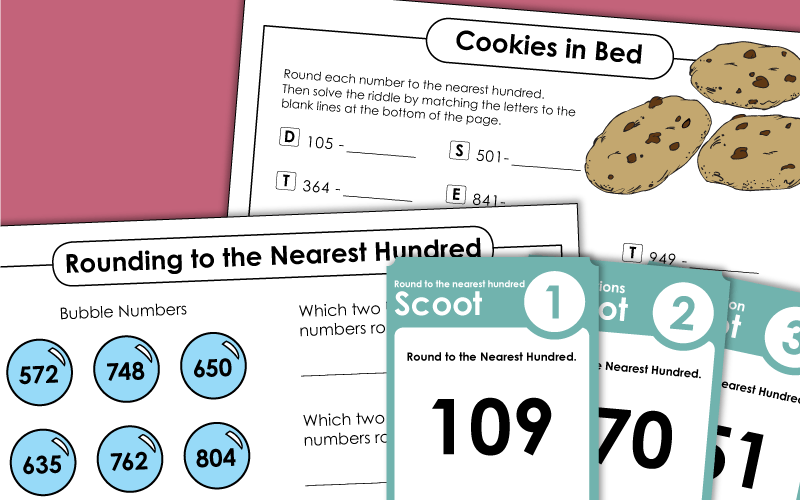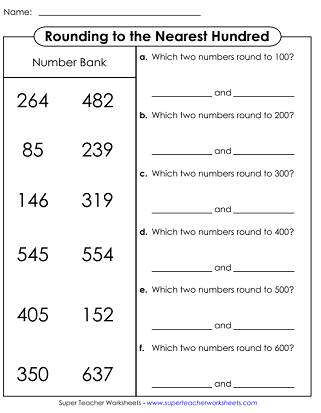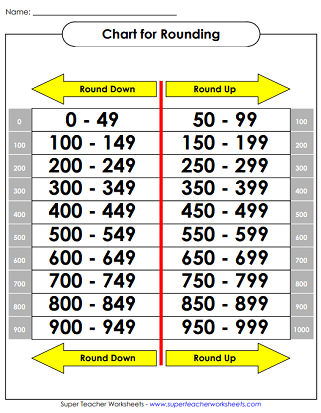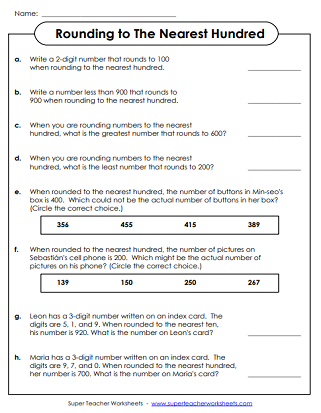# Rounding to the Nearest Hundred

Download and print files on Rounding to the Nearest Hundred. Includes a Scoot game, printable lessons, a math riddle worksheet, a foldable fortune teller, and several worksheets.## Nearest Hundred(3-Digit Numbers Only)

Part 1: Round to the nearest hundred. Part 2: Bubble numbers. Part 3: Web. Part 4: True and false.
Round up and down for each number. Circle the number that is rounded to the nearest hundred. Includes only three-digit numbers.
This worksheet has higher-order thinking questions to use with your rounding lesson.
Help the rockets land on the correct planets by rounding the 2-digit numbers to the nearest 10.
In this printable math activity, round to the nearest one hundred to solve the rounding caterpillars.
In this fast-paced card game, students move around the classroom to desks with rounding task cards attached to them. For each card, they round the given number to the nearest hundred.
Print out eight pages of task cards and cut them apart. Each one has a 3-digit number that students must round. This file can be used for classroom games, learning centers, or small group instruction.
Students build an origami cootie catcher (aka fortune teller). Then they use it to play a learning game in which they round numbers to the nearest hundred.
Choose numbers from the bank that round the the numbers given.

## Rounding Tables / Charts

Use this thousands chart to round numbers to the nearest hundred.
This is another version of the thousands chart to help kids round to the nearest hundred.

## Nearest Hundred(3 and 4-Digit Numbers)

Practice rounding these numbers to the nearest hundred.
These task cards have 3 and 4-digit numbers. Round each to the nearest hundred. Use for learning centers, classroom games, and small group instruction.
Round each number to the nearest hundred. Includes 3 and 4-digit numbers. Then use the answers to decode the answer to the riddle: Why did the girl put a cookie under her pillow when she went to bed?
On this PDF you'll have 30 Scoot! cards. Place them on desks around your classroom. Students move from desk to desk rounding the numbers on the cards.
A set of multiple choice questions for rounding to the nearest hundred.
Use the cypher key at the top of the page to decode the 3 and 4-digit numbers. Then round each to the nearest hundred.
Round each number to the nearest hundred and tell whether you're "rounding up" or "rounding down."

## Nearest Tens &Hundreds Mixed

Use the number lines to help round numbers to the nearest 10 and nearest 100.
Answer a set of questions and word problems on rounding numbers.
Round each number to the nearest ten.  Then round to the nearest hundred.
A set of questions for rounding to the nearest ten and hundred.
This file has 30 task cards. Students round 2, 3, and 4-digit numbers to the nearest ten and the nearest hundred.
Rounding (Nearest Ten)

Choose for a large selection of PDF rounding charts, worksheets, and task cards on rounding to the nearest ten.

Rounding (Nearest Thousand)

We also have some worksheets on rounding to the nearest thousand.

Place Value

STW has a mammoth collection of place value worksheets for all levels.

## Rounding Worksheet PicturesMy Account
Site Information